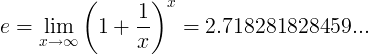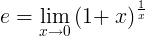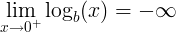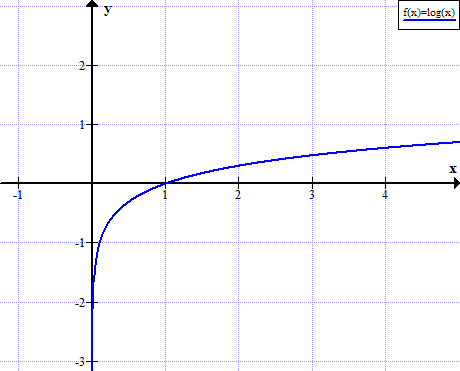# Logarithm free online calculator

Calculate logarithm of a number to any base:

* Use e for scientific notation. E.g: 5e3, 4e-8, 1.45e12

When:

b y = x

Then the base b logarithm of a number x:

logb x = y

## Anti-logarithm calculator

In order to calculate log-1(y) on the calculator, enter the base b (10 is the default value, enter e for e constant), enter the logarithm value y and press the calculate button:

When

y = logb x

The anti logarithm (or inverse logarithm) is calculated by raising the base b to the logarithm y:

x = logb-1(y) = b y

## Logarithm definition

When b is raised to the power of y is equal x:

b y = x

Then the base b logarithm of x is equal to y:

logb(x) = y

For example when:

24 = 16

Then

log2(16) = 4

## Logarithm as inverse function of exponential function

The logarithmic function,

= logb(x)

is the inverse function of the exponential function,

= by

So if we calculate the exponential function of the logarithm of x (x>0),

(-1(x)) = blogb(x) = x

Or if we calculate the logarithm of the exponential function of x,

-1((x)) = logb(bx) = x

## Natural logarithm (ln)

Natural logarithm is a logarithm to the base e:

ln(x) = loge(x)

When e constant is the number:or## Inverse logarithm calculation

The inverse logarithm (or anti logarithm) is calculated by raising the base b to the logarithm y:

x = log-1(y) = b y

### Logarithmic function

The logarithmic function has the basic form of:

(x) = logb(x)

## Logarithm rules

Rule nameRule

#### Logarithm product rule

logb(x ∙ y) = logb(x) + logb(y)

#### Logarithm quotient rule

logb(x / y) = logb(x) - logb(y)

#### Logarithm power rule

logb(y) = y ∙ logb(x)

#### Logarithm base switch rule

logb(c) = 1 / logc(b)

#### Logarithm base change rule

logb(x) = logc(x) / logc(b)

#### Derivative of logarithm

(x) = logb(x) ⇒ f ' (x) = 1 / ( x ln(b) )

#### Integral of logarithm

logb(xdx = x ∙ ( logb(x) - 1 / ln(b) ) + C

#### Logarithm of negative number

logb(x) is undefined when x≤ 0

#### Logarithm of 0

logb(0) is undefinedlogb(1) = 0

logb(b) = 1

#### Logarithm of infinity

lim logb(x) = ∞,when x→∞

See: Logarithm rules

#### Logarithm product rule

The logarithm of the multiplication of x and y is the sum of logarithm of x and logarithm of y.

logb(x ∙ y) = logb(x) + logb(y)

#### For example:

log10(3 ∙ 7) = log10(3) + log10(7)

#### Logarithm quotient rule

The logarithm of the division of x and y is the difference of logarithm of x and logarithm of y.

logb(x / y) = logb(x) - logb(y)

#### For example:

log10(3 / 7) = log10(3) - log10(7)

#### Logarithm power rule

The logarithm of x raised to the power of y is y times the logarithm of x.

logb(y) = y ∙ logb(x)

#### For example:

log10(28) = 8∙ log10(2)

#### Logarithm base switch rule

The base b logarithm of c is 1 divided by the base c logarithm of b.

logb(c) = 1 / logc(b)

#### For example:

log2(8) = 1 / log8(2)

#### Logarithm base change rule

The base b logarithm of x is base c logarithm of x divided by the base c logarithm of b.

logb(x) = logc(x) / logc(b)

For example, in order to calculate log2(8) in calculator, we need to change the base to 10:

log2(8) = log10(8) / log10(2)

#### Logarithm of negative number

The base b real logarithm of x when x<=0 is undefined when x is negative or equal to zero:

logb(xis undefined when x ≤ 0

#### Logarithm of 0

The base b logarithm of zero is undefined:

logb(0) is undefined

The limit of the base b logarithm of x, when x approaches zero, is minus infinity:See: log of zero

#### Logarithm of 1

The base b logarithm of one is zero:

logb(1) = 0

For example, teh base two logarithm of one is zero:

log2(1) = 0

See: log of one

#### Logarithm of infinity

The limit of the base b logarithm of x, when x approaches infinity, is equal to infinity:

lim logb(x) = ∞, when x→∞

See: log of infinity

#### Logarithm of the base

The base b logarithm of b is one:

logb(b) = 1

For example, the base two logarithm of two is one:

log2(2) = 1

#### Logarithm derivative

When

(x) = logb(x)

Then the derivative of f(x):

f ' (x) = 1 / ( x ln(b) )

See: log derivative

#### Logarithm integral

The integral of logarithm of x:

logb(xdx = x ∙ ( logb(x) - 1 / ln(b) ) + C

#### For example:

log2(xdx = x ∙ ( log2(x) - 1 / ln(2) ) + C

## Logarithm approximation

log2(x) ≈ n + (x/2n - 1) ,

## Complex logarithm

For complex number z:

z = re = x + iy

The complex logarithm will be (n = ...-2,-1,0,1,2,...):

Log z = ln(r) + i(θ+2nπ) = ln(√(x2+y2)) + i·arctan(y/x))

#### Problem #1

Find x for

log2(x) + log2(x-3) = 2

#### Solution:

Using the product rule:

log2(x∙(x-3)) = 2

Changing the logarithm form according to the logarithm definition:

x∙(x-3) = 22

Or

x2-3x-4 = 0

x1,2 = [3±√(9+16) ] / 2 = [3±5] / 2 = 4,-1

Since the logarithm is not defined for negative numbers, the answer is:

x = 4

#### Problem #2

Find x for

log3(x+2) - log3(x) = 2

#### Solution:

Using the quotient rule:

log3((x+2) / x) = 2

Changing the logarithm form according to the logarithm definition:

(x+2)/x = 32

Or

x+2 = 9x

Or

8x = 2

Or

x = 0.25

## Graph of log(x)

log(x) is not defined for real non positive values of x:## Logarithms table

xlog10xlog2xlogex
0undefinedundefinedundefined
0+- ∞- ∞- ∞
0.0001-4-13.287712-9.210340
0.001-3-9.965784-6.907755
0.01-2-6.643856-4.605170
0.1-1-3.321928-2.302585
1000
20.30103010.693147
30.4771211.5849631.098612
40.60206021.386294
50.6989702.3219281.609438
60.7781512.5849631.791759
70.8450982.8073551.945910
80.90309032.079442
90.9542433.1699252.197225
1013.3219282.302585
201.3010304.3219282.995732
301.4771214.9068913.401197
401.6020605.3219283.688879
501.6989705.6438563.912023
601.7781515.9069914.094345
701.8450986.1292834.248495
801.9030906.3219284.382027
901.9542436.4918534.499810
10026.6438564.605170
2002.3010307.6438565.298317
3002.4771218.2288195.703782
4002.6020608.6438565.991465
5002.6989708.9657846.214608
6002.7781519.2288196.396930
7002.8450989.4512116.551080
8002.9030909.6438566.684612
9002.9542439.8137816.802395
100039.9657846.907755
10000413.2877129.210340

Logarithm calculator »

Logarithm - log(x) »

Currently, we have around 1975 calculators, conversion tables and usefull online tools and software features for students, teaching and teachers, designers and simply for everyone.